Watson's Blog 2018-12-31T08:04:04.461Z http://ulsonhu.cn/ Pic by W.H Hexo 2018 飘着 http://ulsonhu.cn/2018就飘着.html 2018-12-30T16:00:00.000Z 2018-12-31T08:04:04.461Z 眼看着2018就要结束了，倒不是非写个命题作文，但是整理整理过去一年的感悟还是很值得的。不过也不能就叫年终总结吧，想着今年暑假没能回去，也在外飘了1整年了，所以今年就飘着吧。

## 正经事

• 第一次穿学士服
• 第一次和老外talking
• 第一次写英文工作邮件
• 第一次剪/拍视频
• 第一次吃榴莲
• 第一次用香水
• 第一次座摩天轮
• 第一次做菜

## 不正经事

### 阅读

• 《蒙元入侵前夜的中国日常生活》
这本书背景是蒙古入侵前的南宋都城临安（杭州）的社会生活。在兵临城下的情况下，临安城内如是一如既往的悠闲。其中对衣食住行、婚丧嫁娶习俗和消闲时光的描绘格外有趣。抛开历史环境不谈，是一部描绘古代社会生活难得的参考资料。

• 《月亮与六便士》
很多人即使没有看过小说，应该也听过这个 quote：If you look on the ground in search of a sixpence, you don’t look up, and so miss the moon。书中塑造了一个纯粹的、毫无妥协的艺术家形象，其人物原型的法国画家梵高。我想我不必纠结于斯珠兰抛妻损友的行为的对错，大概艺术与世俗规矩本就水火不相容，对于他来说，生存与毁灭是不存在中间地带的。

• 《刀锋》《人性的枷锁》、《总结：毛姆写作生活回忆》
依然是毛姆的几篇代表作，其中《人性的枷锁》通过描写菲利普的一生，从大家的对话讨论或者内心独白中以不同的视角道出了人在成长过程中对于人生的认识和感悟。我想其中有挺多大概是作者自身的看法吧。并没有像《月亮与六便士》那样喜欢这本书，大概因为主角优柔寡断的性格很是让人讨厌吧，有时候会觉得主角很多时候完全是自讨苦吃，作茧自缚，可是又不得不承认每个人其实也都活在各自的枷锁之中，甚至有时候主角成长过程中的一些可笑的行为大概也能勾起自己曾经经历过的一些磕磕绊绊呢。也许人的一生就是不断地突破自己的一个个枷锁的过程。至于人生的意义，这个大概又太深奥了。从蜉蝣到宇宙，不论在什么尺度下，时间仍然像一条无尽的长河，大概没有什么东西是有绝对意义的吧。《刀锋》比较喜欢里面一些比较犀利的对话和讨论。整体来讲并不如《月亮与六便士》那样喜欢，但是要超过《人性的枷锁》一点。大概我不太懂主角拉里到底追寻的是什么。不过毛姆先生在书里把自己写得也是相当犀利和风趣，和《人性》里那个无知的少年似乎是判若两人。

• 《群上回唱》
高中时读过《追风筝的人》，再读这部《群山回唱》，依然折服于作者高超的叙事能力。

• 《人间失格》
其实作者一直在展示人性黑暗、肮脏的一面，但我想坐着本人是存在精神洁癖的。但是作者毕竟是人，他发现自身也存在肮脏的一面，问题在于，他并没有正视它或做积极的改变，导致这样的心结始终积郁难解，最终也只有走向人间失格。但是，看完这样一部文学作品，我想反求诸己总是可行的，爱会让一切变好。就像陀思妥耶夫斯基，借那么长的一步《卡拉马佐夫兄弟》，给读者传达出，坚信“善”的力量。

• 《萤火虫小巷》、《岛上书店》
两本都是来自美国作家的畅销书，没有什么太值得说的。作者讲述的故事本身是关于友情、亲情与爱情的，读来算事比较温馨。

• 《百年孤独》
读者经常会被开篇第一句话“多年以后，面对行刑队，奥雷里亚诺·布恩迪亚上校将会回想起父亲带他去见识冰块的那个遥远的下午”所吸引。伟大的作品往往第一句就不平凡，百年孤独就是这样一部书。我经常觉得真正的文学需要引导读者自己去探寻人的本质。马尔克斯讲述奥雷里亚诺家族一百多年来，六代人的权利情欲交织起来的家族盛衰史。最后一个儿子生下来就被蚂蚁啃食掉，预言手稿随着马孔多一起消失，展现了一个光怪陆离的世界。其实，越是奇怪、原始，则越是接近人的本质状态。其次，小说的描写，画面感极强，展现了南美洲缤纷多彩的文化。让我对“拉美文学大爆炸”时期的作家，产生浓厚的兴趣。

• 《查泰莱夫人的情人》
大概类似于中国的《金瓶梅》，其实都表达一个反世俗的主题。书中有大段情色描绘，并不淫秽，反而是一种健康的情欲，让读者能够将性和爱分开来看待。想想古代，牡丹亭都被列为禁书，而今，牡丹亭却成了中文系必修的课程。现在的少年们如何排解苦闷，可能就直接看A片了，岂不直接。人大概是欲望的综合体，合理分配吧。

• 《连城诀》
怀念金庸先生去世后读，《连城诀》大概是金庸武侠作品里最黑暗的一个吧。还记得当天消息出来后，赶忙去蔡澜先生的微博下去求证，结果看蔡澜早晨刚发一篇游记，与平常别无二致。尽管知道他们私交很好，但是能做到这么豁达，还是钦佩不已的。

• 《阿莱夫》
博尔赫斯的作品初看时总觉得散乱、难以理解。但是渐渐的，会被他文字传递出的精准性、深刻性所折服。所以，博尔赫斯的小说不读，很难说自己懂近现代的小说。

• 《情人》
懒得长篇大论的谈，可是不长篇大论有说不清杜拉斯的文学风格，两难啊。

• 《野性的呼唤》
拟人化的写法，讲的是一只狗重回野性，回归山林的过程。小说不长，很好读。

• 《红楼梦》
老实说，我还是第一次读红楼梦，对这部经典名著还是抱着敬畏之心的。就像图斯托耶夫斯基的《卡拉马佐夫兄弟》，到今天我也没读到一半。但是，红楼梦让我一读就停不下来，曹雪芹写的每个人都性格迥异。你很难想象一个作者能在一部作品同时塑造出那么多鲜活的人物，有人说，”拿出红楼梦中任意一段对话，你就能知道这是谁说的话。”由此可见，曹雪芹对人物刻画之精准。其实，在我这样一个年纪，读红楼梦大概已经不再单纯只读宝黛间的爱情了。更多的是体会到曹雪芹借大观园这座“青春王国”，传递给读者的人生盛衰相依的道理。也感慨如花美眷，似水流年。

• 《巴黎评论》作家访谈一|二|三

### 谈谈戏曲## 结语

2019年不做计划，只希望能多思考，多踏实干事。

]]>
2018年终感悟
Books & Moive in 2018 http://ulsonhu.cn/new_year_2018.html 2018-12-20T16:00:00.000Z 2018-12-19T15:16:15.937Z

### 读书（文学类）

• 《守望的距离》.周国平
• 《蒙元入侵前夜的中国日常生活》.谢和耐
• 《月亮与六便士》.威廉·萨默塞特·毛姆
• 《刀锋》.威廉·萨默塞特·毛姆
• 《总结：毛姆写作生活回忆》.威廉·萨默塞特·毛姆
• 《人性的枷锁》.威廉·萨默塞特·毛姆
• 《群上回唱》.卡勒德·胡赛尼
• 《我是猫》.夏目漱石
• 《我脑袋里的怪东西》.奥尔罕·帕慕克
• 《人间失格》.太宰治
• 《萤火虫小巷》.克莉丝汀汉娜
• 《浮世画家》.石黑一雄
• 《百年孤独》.马尔克斯
• 《米格尔街》.奈保尔
• 《查泰莱夫人的情人》.DH劳伦斯
• 《岛上书店》.加布瑞埃泽拉文
• 《连城诀》.金庸
• 《阿莱夫》.博尔赫斯
• 《自深深处》.奥斯卡王尔德
• 《情人》. 杜拉斯
• 《野性的呼唤》. 杰克伦敦
• 《红楼梦》.曹雪芹
• 《巴黎评论》作家访谈

### 读书（自我提高及工具书）

• 《Tensorflow机器学习指南》
• 《MySQL必知必会》
• 瑞·达里奥《原则》

• Johnson. 实用多元统计分析.
• 茆诗松. 高等数理统计
• Nasrabadi, Nasser M. “Pattern recognition and machine learning.”

### 看剧

• 《1公升的眼泪》- 看这部剧，的确很戳人泪点。疾病使人绝望，但爱让希望不灭
• 《寻梦环游记》
• 《时空恋旅人》 - 被我当作爆米花电影来看
• 《星际穿越》 - 同被我当作爆米花电影多次翻看
• 《火星救援》 - 近年来的硬科幻佳作
• 《镰仓物语》 - 治愈系佳作2018
• 《JoJo的奇妙冒险》全系列 - Jump动漫化大作，感受荒木老师构造的力与美的世界

]]>
The Times They Are A-Changing
《红楼梦》Dream of the Red Chamber http://ulsonhu.cn/红楼梦-Dream of the Chamber.html 2018-06-23T16:00:00.000Z 2018-12-19T14:19:17.984Z 曹雪芹在红楼梦第五回，借宝玉神游太虚幻境，道出书中女子各自的命运结局，虽已告知告知宝玉众人命运结局，当局者亦浑然不觉，只能看着剧中人一步一步走向那个已经注定的宿命。想罢，人生亦如是，命运总是在可解与不可解之间徘徊。

• 晴雯
霁月难逢，彩云易散。
心比天高，身为下贱。
风流灵巧招人怨。寿夭多因毁谤生，多情公子空牵念。
宝玉看了，又见后面画着一簇鲜花，一床破席，也有几句言词，写道是：
• 袭人
枉自温柔和顺，空云似桂如兰；
堪羡优伶有福，谁知公子无缘。
宝玉看了不解。遂掷下这个，又去开了副册厨门，拿起一本册来，揭开看时，只见画着一株桂花，下面有一池沼，其中水涸泥干，莲枯藕败，后面书云：
• 香菱
根并荷花一茎香，平生遭际实堪伤。
自从两地生孤木，致使香魂返故乡。
• 林黛玉、薛宝钗
可叹停机德，堪怜咏絮才。
玉带林中挂，金钗雪里埋。
宝玉看了仍不解。待要问时，情知他必不肯泄漏；待要丢下，又不舍。遂又往后看时，只见画着一张弓，弓上挂着香橼。也有一首歌词云：
• 元春
二十年来辨是非，榴花开处照宫闱。
三春争及初春景，虎兕相逢大梦归。
后面又画着两人放风筝，一片大海，一只大船，船中有一女子掩面泣涕之状。也有四句写云：
• 探春
才自精明志自高，生于末世运偏消。
清明涕送江边望，千里东风一梦遥。
后面又画几缕飞云，一湾逝水。其词曰：
• 史湘云
富贵又何为，襁褓之间父母违。
展眼吊斜晖，湘江水逝楚云飞。
后面又画着一块美玉，落在泥垢之中。其断语云：
• 妙玉
欲洁何曾洁，云空未必空。可怜金玉质，终陷淖泥中。后面忽见画着个恶狼，追扑一美女，欲啖之意，其书云：
• 迎春
子系中山狼，得志便猖狂。
金闺花柳质，一载赴黄粱。
后面便是一所古庙，里面有一美人在内看经独坐。其判云：
• 惜春
勘破三春景不长，缁衣顿改昔年妆。
可怜绣户侯门女，独卧青灯古佛旁。
后面便是一片冰山，上面有一只雌凤。其判曰：
• 王熙凤
凡鸟偏从末世来，都知爱慕此生才。
一从二令三人木，哭向金陵事更哀。
后面又是一座荒村野店，有一美人在那里纺绩。其判云：
• 巧姐
势败休云贵，家亡莫论亲。
偶因济刘氏，巧得遇恩人。
后面又画着一盆茂兰，旁有一位凤冠霞帔的美人。也有判云：
• 李纨
桃李春风结子完，到头谁似一盆兰。
如冰水好空相妒，枉与他人作笑谈。
后面又画着高楼大厦，有一美人悬梁自缢。其判云：
• 秦可卿
情天情海幻情身，情既相逢必主淫。
漫言不肖皆荣出，造衅开端实在宁。

]]>

## 基础概念

### 2. 第二类Stiring数：

$n$ 个人分成 $k$ 组的分组方案的数量。$$S(n,k) = \frac{1}{k!}\sum_{j=1}^{k}(-1)^{k-j}C_k^j j^n$$$S(n,n-1)=C_n^2=\frac{n(n-1)}{2}$

## 球盒模型

### 标准模型

SeqBallBoxAmt in Box
1Non-DiffMarkNo-limit
2Non-diffMarkNo empty box
3Non-diffUnmarkNo-limit
4Non-diffUnmarkNo empty box
5DiffMarkNo-limit
6DiffMarkNo empty box
7DiffUnmarkNo-limit
8DiffUnmarkNo empty box

### 简例 - 2

1. 8个相同的球放进3个相同的盒子里，每盒至少一个，有几种方法

1. 8个相同的球放进3个不同的盒子里，每盒至少一个，有几种方法

1. 8个不同的球放进3个相同的盒子里，每盒至少一个，有几种方法

1. 8个不同的球放进3个不同的盒子里，每盒至少一个，有几种方法

4问中的966种情况，每种情况的三个元素都是互异的，比如 116（因为球是不同的），这三个元素进行全排列$P_3^3=6$, 乘以 966=5796 即为所求

1. 8个相同的球放进3个相同的盒子里，有几种方法

1. 8个相同的球放进3个不同的盒子里，有几种方法
预先在三个盒子种各放入一小球，则问题转化为11同球放3不同盒子，每盒至少1个，几种方法？ 用插板法，$C_10^2=45$

2. 8个不同的球放进3个不同的盒子里，有几种方法

1. 8个不同的球放进3个相同的盒子里，有几种方法

(7问) 中的一般情况(3个元素都相异)，比如116，一共有6种排列(球是不同的)，此问中，盒子是相同的，因此这6种排列都只算一种情况。 但如果2个元素相同的时候，有且只有008，只有3种排列，我们多添加3种进去，令其也重复6次，则6561+3就是 所有的情况都重复了6次，$\frac{6561+3}{6}=1094$ 即为所求。

## 匹配问题

$$P(A_1)=\frac{(n-1)!}{n!} = \frac{1}{n}$$

$P(A_2)=P(A_3)=\cdots =P(A_n)=P(A_1)$，所以 $S_1=n\cdot \frac{1}{n}=1$

$$P(B) = 1-\frac{1}{2!}+\frac{1}{3!}-\cdots +(-1)^{n-1}\frac{1}{n!}$$

$$1-e^{-1} = 1-\frac{1}{2!}+\frac{1}{3!}-\cdots +(-1)^{n-1}\frac{1}{n!}+\cdots = \sum_{n=1}^{\inf}\frac{(-1)^{n-1} }{n!}$$

]]>

Schwarz不等式知识归纳 http://ulsonhu.cn/Schwarz不等式知识归纳.html 2018-06-09T16:00:00.000Z 2018-07-04T14:30:15.741Z Schwarz 不等式是个应用广泛的不等式，常见于线性代数的内积空间，数学分析的无穷级数，连续函数的积分以及概率论中的方差、协方差。

$\vert \langle x,y\rangle \vert ^2 \leq \langle x,x\rangle \dot \langle y,y \rangle$

$$\vert \int f^*(x)g(x)dx \vert ^2 \leq \int \vert f(x) \vert ^2dx \dot \int \vert g(x) \vert ^2dx$$

$$\vert \int_0^1 \bar{f(t)}g(t) dt \vert^2 \leq \int_0^1 \vert f(t) \vert ^2dt \dot \int_0^1 g(t) \vert ^2dt$$

$$d(\mathbf{x},\mathbf{y})=\vert \mathbf{x}-\mathbf{y} \Vert = \sqrt{\langle\mathbf{x}-\mathbf{y},\mathbf{x}-\mathbf{y} \rangle}$$

1. $d(\mathbf{x},\mathbf{y})=d(\mathbf{y},\mathbf{x})$
2. $d(\mathbf{x},\mathbf{y})\geq 0$ ，当且仅当 $\mathbf{x}=\mathbf{y}$时，$d(\mathbf{x},\mathbf{y})=0$。
3. $d(\mathbf{x},\mathbf{y})\leq d(\mathbf{x},\mathbf{z})+d(\mathbf{z},\mathbf{y})$

### 延伸点

• Schwarz不等式
• Jesen不等式（凸分析与随机数学中出现得比较多）
• 赫尔德（Holder）不等式
• 闵可夫斯基（Minkowski）不等式
• Hilbert空间的贝塞尔不等式
• Poincare不等式（变分学中非常重要的不等式）
• Soblev空间嵌入定理（在变分学和偏微分方程中非常重要的不等式）

• Banach不动点定理
• Hilbert空间的投影定理
• Hahn-Banach定理（核心中的核心）与分离超平面定理
• 反函数定理和隐函数定理（赋范线性空间的微分学）
• 阿尔采拉-阿斯科利定理
• Sobolev嵌入定理
• Rellich Kontracheev紧嵌入定理
• Eblerlin Schulyman定理
• Lagrange乘子定理
• Kuhn-Tucker定理（无限维空间的版本，基于Hahn-Banach定理）
• Pontryagin最大值原理（利用Frechet微分理论和前面的乘子定理去理解即可）
• Brouwer（布劳威尔）不动点定理
• Schaulder（绍德）不动点定理

### 复变函数中的柯西不等式

$$\vert f^{(n)}(z_0) \vert \leq \frac{n!M}{R^n}$$

### 其它推广

$$\sqrt {\sum _{i=1}^{n} (\sum _{j=1}^{m} a_{ij})^2} \leq \sum _{j=1}^{m} \sqrt {\sum _{i=1}^{n}a_{ij}^2} \\ m \req \alpha > 0,(\sum _{i=1}^{n} \prod _{j=1}^{m} a_{ij})^{\alpha }\leq \prod _{j=1}^{m}\sum _{i=1}^{n} a_{ij}^\alpha$$

]]>
Schwarz 不等式是个应用广泛的不等式，常见于线性代数的内积空间，数学分析的无穷级数，连续函数的积分以及概率论中的方差、协方差。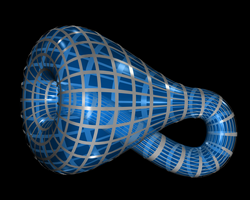• $K$ 是有界闭集
• $K$ 的任意无限子集必存在极限点
• $K$ 中的任意序列必有收敛子列
• $K$ 的任意开覆盖必有有限子覆盖

• 首先当然是要给出拓扑空间紧性的定义。
• 接下来当然是会举一些例子，一方面是把枯燥的定义从抽象中拉回来，另一方面也是非常重要的是给出紧空间的存在性的证据，因为定义总是可以随便给的，这样子我可以给出具有任意优良性质的定义来，然而所定义的东西如果是不存在的话，相关的一切性质其实都是空谈。
• 然后我们将介绍从已有的紧空间构造新的紧空间的方法：包括集合的交、并、补，以及子空间、商空间和积空间——这一系列都是标准套路。在这里将会出现一个大定理，就是刚才提到的 $\text{Tychonoff}$ 定理。
• 接下来将暂时中断一下，讨论一下稍微具体一点的度量空间(Metric Space)中的紧性。因为度量空间更加具体一些，所以能得到的性质也更丰富一些。
• 最后我们将简要介绍一些将非紧空间（non-compact space）转化为紧空间（compactification，紧化）的初步知识。

• 任意由有限点集所构成的拓扑空间是紧的。因为无论在它上面给怎么样的拓扑，它所有的开集的个数总是有限的，所以任意开覆盖本身就是有限覆盖了。
• 具有余有限拓扑（cofinite topology ）的空间是紧的。因为假设 $\mathcal{A}$ 是具有 cofinite topology 的空间 $X$ 的一个开覆盖，从 $\mathcal{A}$ 中任选一个非空的元素 $A_0$，由 cofinite topology 的定义，知道 $X-A_0$ 只有有限个元素 $x_1,\ldots,x_n$ ，对于每一个 $x_i$，$i=1,\ldots,n$ 可以找到一个 $A_i\in\mathcal{A}$ 使得 $x_i\in A_i$ ，这样，${A_0,A_1,\ldots,A_n}$ 就是 $X$ 的开覆盖 $\mathcal{A}$ 的一个有限子覆盖。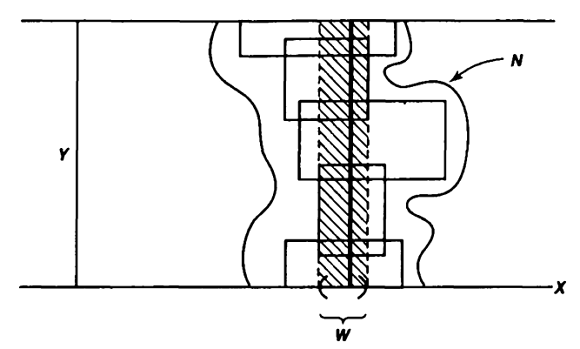• 基开覆盖，如果每个 $A_\lambda\in\mathcal{A}$ 属于 $X$ 的某个给定的拓扑基；
• 子基开覆盖，如果每个 $A_\lambda\in\mathcal{A}$ 属于 $X$ 的某个给定的子基拓扑。

• 闭基，如果 ${X-B|B\in\mathcal{B}}$ 是 $X$ 的一个基；
• 闭子基，如果 ${X-B|B\in\mathcal{B}}$ 是 $X$ 的一个子基。

• (1)$X$ 是紧的；
• (2)$X$ 的任一基开覆盖有有限子覆盖；
• (3)$X$ 的任意一族满足有限交性质的闭基集合的交非空。

• (1)$X$ 是紧的；
• (2)$X$ 的任意子基开覆盖有有限子覆盖；
• (3)$X$ 的任意一族满足有限交性质的闭子基集合有非空的交。

• (1) $\Rightarrow$ (2) 显然。
• (2) $\Leftrightarrow$ (3) 证明和定理 10 的证明完全类似。
• 接下来我们不直接证明 (3) $\Rightarrow$ (1) ，而是证明由本定理的 (3) 可以得到定理 11 的 (3) 。设 $\mathcal{B}={B_\lambda|\lambda\in\Lambda}$ 是 $X$ 中任意一族满足有限交性质的闭基集合，我们要证明它们的交非空。

$\mathcal{C}$ 的每个元素是一个闭集集合，由于每个基集合都是有限个子基集合的交，我们有每个闭基集合都是有限个闭子基集合的并。即 $\forall C_\mu \in \mathcal{C}, \mu\in M$$C_\mu = S_1\cap\cdots\cap S_n$$这里为了避免下标爆炸，只好乱用一下符号了。实际上对于每个不同的$\mu$，$n$是不一样的，而且$S_1$到$S_n$也可能是不同的集合。接下来的下标也会有点乱……现在我们考虑某个特定的$\mu$，如果我们能证明至少存在一个$i$使得$S_i\in\mathcal{C}$，那么对于每个闭基集合$C_\mu, \mu\in M$，取对应的那个$S_i$组成一个集族${S_\mu}\subset \mathcal{C}$，因此它满足有限交条件，由 (3) 的条件知（注意每个$S_\mu$是闭子基集合），它们的交非空。由此可以立即得到：集族$\mathcal{C}$的交也非空。 最后我们就来证明至少存在一个$i$使得$S_i\in\mathcal{C}$，这里终于要用到$\mathcal{C}$的极大性了。用反证法，假设对任意的$i=1,\ldots,n$都有$S_i\not\in\mathcal{C}$。由于闭子基集合同时也是闭基集合，对于每一个$i=1,\ldots,n$， 由$\mathcal{C}$的极大性，知道$$\mathcal{C}\subsetneq\left(\mathcal{C}_i=\{S_i\}\cup \mathcal{C}\right)$$其中$\mathcal{C}_i$必定不能满足有限交条件，亦即，存在$\mathcal{C}_i$的有限子集族$\mathcal{C}’_i$（显然$S_i$包含在其中），其交为空集。将所有这些（有限个）$S_i$并起来，我们得到$C_\mu \in \mathcal{C}$，而将对应的$\mathcal{C}’_i$交起来，我们得到一个$\mathcal{C}$的子集族$$\mathcal{C}_f=\bigcap_{i=1}^n\mathcal{C}’_i$$由刚才的构造，知道$$C_\mu\cup\left(\bigcap_{C\in\mathcal{C}_f}C\right)=\emptyset$$ 而$\{C_\mu\}\cup \mathcal{C}_f$是$\mathcal{C}$的一个有限子集族，这与$\mathcal{C}$满足有限交条件相矛盾。证完。 有了这些准备工作之后，Tychonoff 的证明也就变得简单了： 定理 9 的证明：空间$\prod_{\lambda}X_\lambda$的一族子基是$$\{p^{-1}_\lambda(V_\lambda)|V_\lambda\subset X_\lambda, V_\lambda\text{open},\lambda\in \Lambda\}$$因此对应的闭子基的每个元素集合是形状如$$\prod_{\lambda\in\Lambda}C_\lambda$$的集合，其中$C_\lambda$是$X_\lambda$中的闭集（实际上除了最多一个之外，其他的全都是整个空间$X_\lambda$）。对于任意一族如上形式的闭子基集族$\mathcal{A}$，如果它们满足有限交性质，我们证明它们的交非空即可。根据上面的形式可以知道，将$\mathcal{A}$以自然投影投影到任意的$X_\lambda$中，仍然得到一族满足有限交性质的闭集，由$X_\lambda$本身的紧性，知道它们的交非空，因此可以选择其中一点$x_\lambda$。所有的这些点就构成了$\prod_\lambda X_\lambda$中$\bigcap_{A\in\mathcal{A}}A$里的一点$(x_\lambda)_{\lambda\in\Lambda}$，即证。 最后，闲聊一下文章标题图片 Klein Bottle ，课堂上提到拓扑必然会举到的例子，什么橡皮泥啊、克莱因瓶之类的。默比乌斯带还是可以理解的，因为可以做出实物来，但是当时一直觉得克莱因瓶是不对的，所谓顺着瓶子的壁可以从里面爬到外面，我看这各种克莱因瓶的图，都觉得是不对的。到现在终于知道为什么了——确实是不对的，不过不是说 Klein Bottle 不存在，如果用 schematic representation 的话，还是比较可以接受的，只是关于 Klein Bottle 的 visualization ，却是有问题的，因为 Klein Bottle 不能嵌入到$\mathbb{R}^3$中，直观地来说，我们无法在我们生活的三维世界里造出一个克莱因瓶来，如果强行把它放到$\mathbb{R}^3$中，就会出现自相交了，也就是平时所看到的那些图里明显的不对劲的地方了。 不过我比较奇怪，其实用图片来做 visualization 的话，实际上是在$\mathbb{R}^2$中了，结果最大的问题还是出在人类的想象力上吗？不过我觉得这里贴的这张图似乎有点意思了——因为看不太出那么明显的自交。 ]]> 关于空间的紧性，我们在之前的分析中已经见过了：例如在实数轴上的有界闭区间就是典型的紧集，紧集具有很多优良的性质，比如我们知道在有界闭区间上的连续函数一定是一致连续的，并且能取到最大值和最小值。 Note-关于事件的运算 http://ulsonhu.cn/Note-关于事件的运算.html 2018-04-19T16:00:00.000Z 2018-05-04T14:59:25.393Z 关于事件的运算 $$\underset{n \rightarrow \infty}{\overline{lim}} A_n= \bigcap _{k=1}^{\infty} \bigcup _{n=k}^{\infty} A_n$$ $$\underset{n \rightarrow \infty}{\underline{lim}} A_n= \bigcup _{k=1}^{\infty} \bigcap _{n=k}^{\infty} A_n$$ 其中，$\underset{n \rightarrow \infty}{\overline{lim}} A_n​$为事件序列$\{A_n\}​$的上限事件，表示$A_n​$发生无穷多次。类似的，称$\underset{n \rightarrow \infty}{\underline{lim}} A_n​$为事件序列的下限事件，表示$A_n​$之多只有有限个不发生。 显然，有$$\underset{n \rightarrow \infty}{\overline{lim}} A_n \supset \underset{n \rightarrow \infty}{\underline{lim}} A_n$$ 特别的，当$\underset{n \rightarrow \infty}{\overline{lim}} A_n = \underset{n \rightarrow \infty}{\underline{lim}} A_n$时，记$ \underset{n \rightarrow \infty}{lim} A_n \equiv \underset{n \rightarrow \infty}{\overline{lim}} A_n = \underset{n \rightarrow \infty}{\underline{lim}} A_n$，并称它为事件序列$\{A_n\}$的极限事件。 由德摩根定律，有 $$\overline{(\bigcap _{k=1}^{\infty} \bigcup _{n=k}^{\infty} A_n)}=\bigcup _{k=1}^{\infty} \bigcap _{n=k}^{\infty} \overline{A_n}$$ $$\overline{(\bigcup _{k=1}^{\infty} \bigcap _{n=k}^{\infty} A_n)}=\bigcap _{k=1}^{\infty} \bigcup _{n=k}^{\infty} \overline{A_n}$$ 因此$$\underset{n \rightarrow \infty}{\underline{lim}} \overline{A_n} = \overline{(\underset{n \rightarrow \infty}{\overline{lim}} A_n)}$$$$\underset{n \rightarrow \infty}{\overline{lim}} \overline{A_n} = \overline{(\underset{n \rightarrow \infty}{\underline{lim}} A_n)}$$ 由此引出的$\text{Borel-Cantelli}$引理，在概率论中有着众多应用。$\text{Borel-Cantelli lemma}$: 1. 若随机事件序列$\{A_n\}$满足$$\sum_{n=1}^{\infty}P(A_n)<\infty$$则$$P\{ \underset{n \rightarrow \infty}{\overline{lim}} A_n\}=0, P\{ \underset{n \rightarrow \infty}{\underline{lim}} \overline{A_n}\}=1$$ 2. 若$\{A_n\}$是相互独立的随机事件序列，则$$\sum_{n=1}^{\infty}P(A_n)=\infty$$的充要条件为 $$P(\underset{n \rightarrow \infty}{\overline{lim}} A_n)=1 \text{ or } P(\underset{n \rightarrow \infty}{\underline{lim}} \overline{A_n})=0 ​$$ 由此，我们可以进一步讨论以概率1收敛. $$\xi_n(\omega) \overset{a.s.}{\rightarrow} \xi (\omega)$$ ]]> Note系列为日常笔记。 接触向量自回归模型 http://ulsonhu.cn/接触向量自回归模型.html 2018-04-13T16:00:00.000Z 2018-05-04T14:58:37.115Z VAR方法通过把系统中每一个内生变量,作为系统中所有内生变量的滞后值的函数来构造模型，从而回避了结构化模型的要求。Engle, Granger（1987）指出两个或多个非平稳时间序列的线性组合可能是平稳的。假如这样一种平稳的或的线性组合存在，这些非平稳（有单位根）时间序列之间被认为是具有协整关系的。这种平稳的线性组合被称为协整方程且可被解释为变量之间的长期均衡关系。VAR模型对于相互联系的时间序列变量系统是有效的预测模型，同时，向量自回归模型也被频繁地用于分析不同类型的随机误差项对系统变量的动态影响。如果变量之间不仅存在滞后影响，而不存在同期影响关系，则适合建立VAR模型，因为VAR模型实际上是把当期关系隐含到了随机扰动项之中。 ## 目录 • 定义 • 概述 • 平稳性检验 • 向量自回归模型（VAR） • E-G检验与JJ检验 • 误差修正模型 • 脉冲响应函数 • 方差分解 ## 定义 向量自回归模型（英语：Vector Autoregression model，简称VAR模型）是一种常用的计量经济模型，由C.Sims提出。它扩充了只能使用一个变量的自回归模型(AR(1))，使容纳大于1个变量，因此经常用在多变量时间序列模型的分析上。 VAR模型描述在同一样本期间内的$n$个变量（内生变量）可以作为它们过去值的线性函数。 一个$VAR§$模型可以写成为： $$y_{t}=c+A_{1}y_{t-1}+A_{2}y_{t-2}+\cdots +A_{p}y_{t-p}+e_{t},$$ 其中：$c$是$n* 1$常数向量，$A_i$是$n*n$矩阵。$e_t$是$n*1$误差向量，满足： 1.$E(e_t)=0$,误差项的均值为$0$2.$E(e_{t}e_{t}’)=\Omega $, 误差项的协方差矩阵为$\Omega$.（一个$n*n$正定矩阵） 3.$E(e_{t}e_{t-k}’)=0$, （对于所有不为$0$的$k$都满足）,误差项不存在自相关 ### 结构向量自回归 一个结构向量自回归（Structural VAR）模型可以写成为： $$B_{0}y_{t}=c_{0}+B_{1}y_{t-1}+B_{2}y_{t-2}+\cdots +B_{p}y_{t-p}+\epsilon _{t},$$ 其中：$c_0$是$n*1$常数向量，$B_i$是$n*n$矩阵，$\epsilon_t$是$n*1$误差向量。 ## 概述 简单说VAR模型建立 第一步：不问序列如何均可建立初步的VAR模型（建立过程中数据可能前平稳序列，也可能是部分平稳，还可能是没协整关系的同阶不平稳序列，也可能是不同阶的不平稳序列，滞后阶数任意指定。所有序列一般视为内生向量)， 第二步：在建立的初步VAR后进行 1. 滞后阶数检验，以确定最终模型的滞后阶数 2. 在滞后阶数确定后进行因果关系检验，以确定哪些序列为外生变量 至此重新构建VAR模型（此时滞后阶数已定，内外生变量已定），再进行AR根图表分析， • 如单位根均小于1，VAR构建完成可建立误差修正模型、脉冲响应函数及方差分解。 • 如单位根有大于1的，考虑对原始序进行降阶处理（一阶单整序列处理方法：差分或取对数，二阶单整序列：理论上可以差分与取对数同时进行，但由于序列失去了经济含义，应放弃此处理，可考虑序列的趋势分解，如分解后仍然不能满足要求，可以罢工，不建立任何模型，休息或是打砸了电脑），处理过后对新的序列（包括最初的哪些平稳序列）不断重复第一步与第二步，直至满足稳定性为止 第三步，建立最终的VAR后，可考虑SVAR模型。如果变量不仅存在滞后影响，还存在同期影响关系，则建立VAR模型不太合适，这种情况下需要进行结构分析。 ## 平稳性检验 平稳性检验有3个作用： 1. 检验平稳性，若平稳，做格兰杰检验，非平稳，作协整检验。 2. 协整检验中要用到每个序列的单整阶数。 3. 判断时间序列的数据生成过程。 VAR建模时lag intervals for endogenous要填滞后期，但是此时你并不能判断哪个滞后时最优的，因此要多次尝试，选择不同的滞后期，至$AIC$或$SC$最小时，所对应着的滞后为最优滞后，此时做出来的VAR模型才较为可靠。 协整检验前需要提前估计VAR模型，原因在于，协整检验是对滞后期和检验形式非常敏感的检验，首先需要确定最优滞后。由于VAR是无约束的，而协整是有约束的，因此协整检验的最优滞后一般为VAR的最优滞后减去1，确定了最优滞后后，再去诊断检验形式，最终才能做协整。 ## E-G检验与JJ检验 当检验的数据是非平稳（即存在单位根），并且各个序列是同阶单整（协整检验的前提），想进一步确定变量之间是否存在协整关系，可以进行协整检验，协整检验主要有EG两步法和JJ检验 • EG两步法是基于回归残差的检验，可以通过建立OLS模型检验其残差平稳性 • JJ检验是基于回归系数的检验，前提是建立VAR模型（即模型符合ADL模式），确定最优滞后阶数 ### Granger因果检验 协整说的是变量之间存在长期的稳定关系，这只是从数量上得到的结论，但不能确定谁是因，谁是果。而因果关系检验就是为了解决这个问题。故，如果存在协整关系，如果存在协整可进一步检测格兰杰因果检验，看看某一个变量的先前的变化对另一个变量是否有影响。 ### 步骤 变量$X$是否为变量$Y$的Granger原因，是可以检验的。 检验$X$是否为引起$Y$变化的Granger原因的过程如下： 第一步：检验原假设“$H_0$：X不是引起Y变化的Granger原因”。首先，估计下列两个回归模型：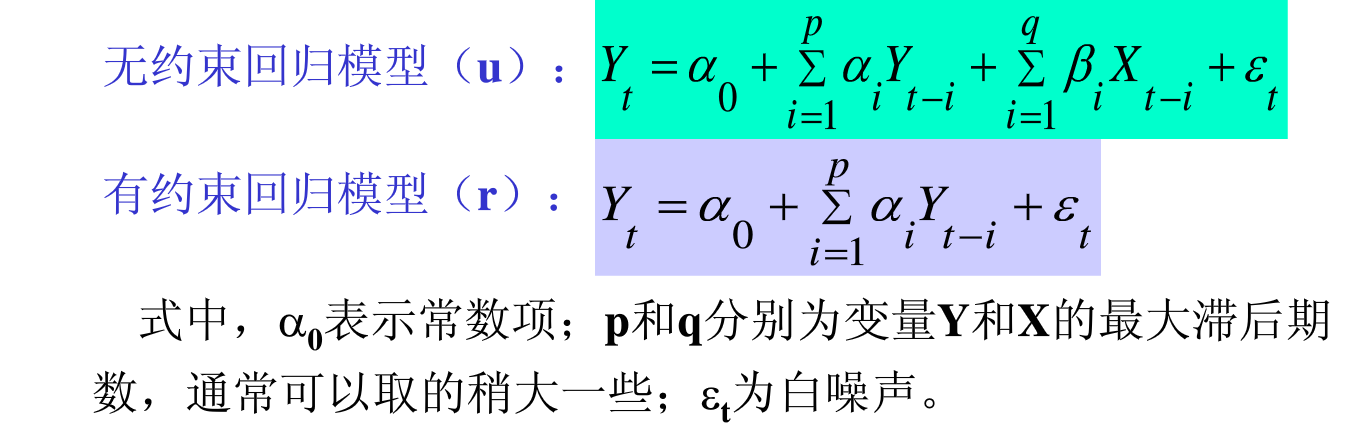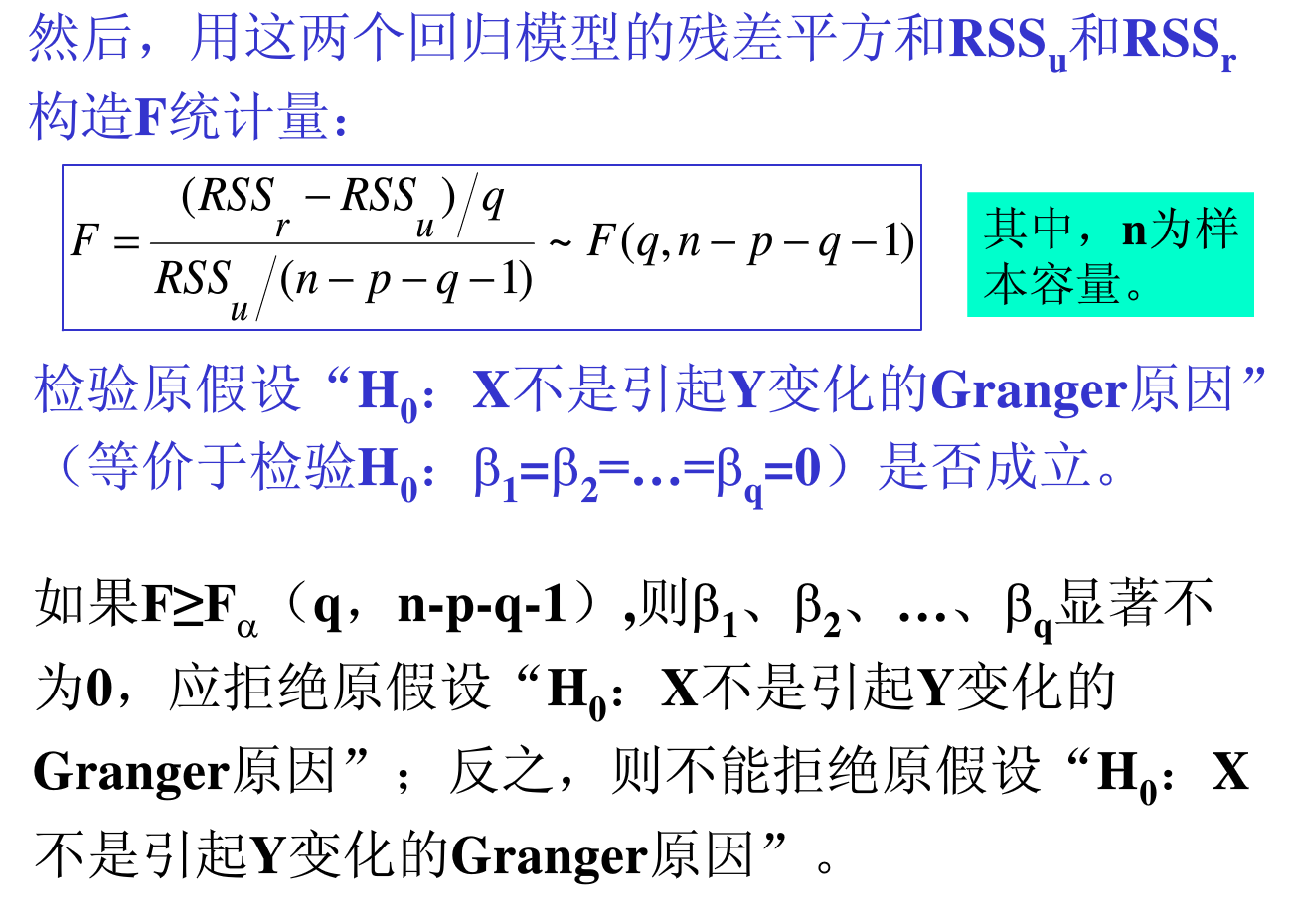第二步：将$Y$与$X$的位置交换，按同样的方法检验原假设“$H_0$：Y不是引起X变化的Granger原因”。 第三步：要得到“$X$是$Y$的Granger原因”的结论，必须同时拒绝原假设“$H_0$：X不是引起Y变化的Granger原因”和接受原假设“$H_0$：Y不是引起X变化的Granger原因”。 格兰杰检验只能用于平稳序列 这是格兰杰检验的前提，而其因果关系并非我们通常理解的因与果的关系，而是说$X$的前期变化能有效地解释$Y$的变化，所以称其为“格兰杰原因”。 ## 误差修正模型（VEC） VEC是有协整约束（即有长期稳定关系）的VAR模型。当变量之间存在协整关系时，可以建立ECM进一步考察短期关系 ## 参考文献 ]]> 向量自回归（VAR,Vector Auto regression）常用于预测相互联系的时间序列系统以及分析随机扰动对变量系统的动态影响。 HMM-隐马尔可夫模型 http://ulsonhu.cn/HMM-隐马尔可夫模型.html 2018-03-31T16:00:00.000Z 2018-04-13T08:55:24.280Z 马尔科夫链 在介绍$\text{HMM}$之前，我们先要了解什么是马尔科夫链。马尔科夫链是一个随机过程，简单来说就是当前时刻的状态与前$p$个时刻的状态有关，即 $$P(z(t) | z(t-1),z(t-2),\cdots,z(1),z(0)) = P(z(t)|z(t-1),z(t-2),\cdots,z(t-p))$$ 这种被称作$p$阶马尔科夫链；为了简化这个过程以方便应用到模型中，我们有两个假设： • 在序列中，当前时刻状态只依赖于前一时刻的状态，也就是$P(z(t)|z(t-1), z(t-2),\cdots, z(1), z(0)) = P(z(t)|z(t-1))$。 • 状态转移的分布不随时间的改变而改变，也就是说任意时刻下的状态产生与时间无关，仅仅与前一状态有关。 但是实际生活中，这种状态本身是很难观测到的，我们只能根据其他的特征来观测这些状态，同时状态之间的 转移矩阵也是很难直接获得的，这就需要隐马尔科夫模型了. ## HMM 形象的例子描述 在引入$\text{HMM}$的公式化描述之前，为了更好的理解 HMM 模型，我们先用一个掷骰子的例子来形象的描述 HMM模型. 模型描述假设我手里有三个不同的骰子。 • 第一个骰子是我们平常见的骰子（称这个骰子为D6），6个面，每个面$（1，2，3，4，5，6）$出现的概率是1/6。 • 第二个骰子是个四面体（称这个骰子为D4），每个面$（1，2，3，4）$出现的概率是1/4。 • 第三个骰子有八个面（称这个骰子为D8），每个面$（1，2，3，4，5，6，7，8）$出现的概率是1/8。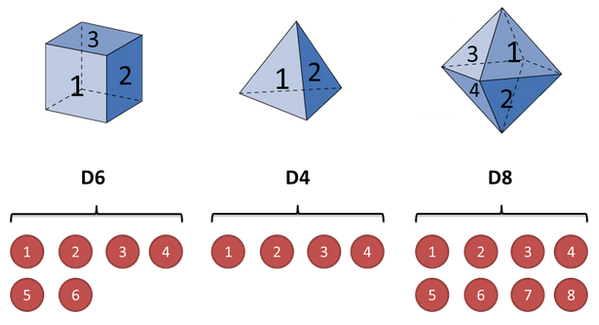假设我们开始掷骰子，我们先从三个骰子里挑一个，挑到每一个骰子的概率都是1/3。然后我们掷骰子，得到一个数字，$1，2，3，4，5，6，7，8$中的一个。不停的重复上述过程，我们会得到一串数字，每个数字都是$1，2，3，4，5，6，7，8$中的一个。例如我们可能得到这么一串数字（掷骰子10次）：$1 6 3 5 2 7 3 5 2 4$这串数字叫做可见状态链。但是在隐马尔可夫模型中，我们不仅仅有这么一串可见状态链，还有一串隐含状态链。在这个例子里，这串隐含状态链就是你用的骰子的序列。比如，隐含状态链有可能是：D6 D8 D8 D6 D4 D8 D6 D6 D4 D8 一般来说，$\text{HMM}$中说到的马尔可夫链其实是指隐含状态链，因为隐含状态（骰子）之间存在转换概率（transition probability）。在我们这个例子里，D6的下一个状态是D4，D6，D8的概率都是1/3。D4，D8的下一个状态是D4，D6，D8的转换概率也都一样是1/3。这样设定是为了最开始容易说清楚，但是我们其实是可以随意设定转换概率的。比如，我们可以这样定义，D6后面不能接D4，D6后面是D6的概率是0.9，是D8的概率是0.1。这样就是一个新的$\text{HMM}$。 同样的，尽管可见状态之间没有转换概率，但是隐含状态和可见状态之间有一个概率叫做输出概率（emission probability）。就我们的例子来说，六面骰（D6）产生1的输出概率是1/6。产生2，3，4，5，6的概率也都是1/6。我们同样可以对输出概率进行其他定义。比如，我有一个被赌场动过手脚的六面骰子，掷出来是1的概率更大，是1/2，掷出来是2，3，4，5，6的概率是1/10。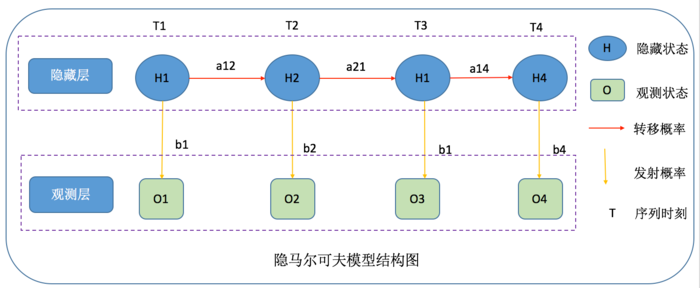隐马尔可夫示意图 其实对于$\text{HMM}$来说，如果提前知道所有隐含状态之间的转换概率和所有隐含状态到所有可见状态之间的输出概率，做模拟是相当容易的。 但是应用$\text{HMM}$模型时候呢，往往是缺失了一部分信息的，有时候你知道骰子有几种，每种骰子是什么，但是不知道掷出来的骰子序列；有时候你只是看到了很多次掷骰子的结果，剩下的什么都不知道。如果应用算法去估计这些缺失的信息，就成了一个很重要的问题。 ## HMM模型解决的三个问题 1. 知道骰子有几种（隐含状态数量），每种骰子是什么（转换概率），根据掷骰子掷出的结果（可见状态链），我想知道每次掷出来的都是哪种骰子（隐含状态链） 这个问题呢，在语音识别领域呢，叫做 解码问题。这个问题其实有两种解法，会给出两个不同的答案。每个答案都对，只不过这些答案的意义不一样。 • 第一种解法求最大似然状态路径，说通俗点呢，就是我求一串骰子序列，这串骰子序列产生观测结果的概率最大。 • 第二种解法呢，就不是求一组骰子序列了，而是求每次掷出的骰子分别是某种骰子的概率。比如说我看到结果后，我可以求得第一次掷骰子是D4的概率是0.5，D6的概率是0.3，D8的概率是0.2 2. 还是知道骰子有几种（隐含状态数量），每种骰子是什么（转换概率），根据掷骰子掷出的结果（可见状态链），我想知道掷出这个结果的概率 看似这个问题意义不大，因为你掷出来的结果很多时候都对应了一个比较大的概率。问这个问题的目的呢，其实是检测观察到的结果和已知的模型是否吻合。如果很多次结果都对应了比较小的概率，那么就说明我们已知的模型很有可能是错的，有人偷偷把我们的骰子給换了 3. 知道骰子有几种（隐含状态数量），不知道每种骰子是什么（转换概率），观测到很多次掷骰子的结果（可见状态链），我想反推出每种骰子是什么(转换概率) 这个问题很重要，因为这是最常见的情况。很多时候我们只有可见结果，不知道$\text{HMM}$模型里的参数，我们需要从可见结果估计出这些参数，这是建模的一个必要步骤 在实际应用中，比如中文分词，我们更多的是利用第三个问题去建模( 当然，如果利用工具的话，你拿到手的时候这部分的参数都是已经训练好了的 )，然后用第一个问题的解码去求隐含状态序列（这也是我们的目标，比如分词、词性标注等）. ### 破解骰子序列 举例来说，我知道我有三个骰子，六面骰，四面骰，八面骰。我也知道我掷了十次的结果1 6 3 5 2 7 3 5 2 4，我不知道每次用了那种骰子，我想知道最有可能的骰子序列 其实最简单而暴力的方法就是穷举所有可能的骰子序列，然后依照第零个问题的解法把每个序列对应的概率算出来。然后我们从里面把对应最大概率的序列挑出来就行了。如果马尔可夫链不长，当然可行。如果长的话，穷举的数量太大，就很难完成了。 另外一种很有名的算法叫做 Viterbi algorithm. ## HMM 的几个要素 经过上面形象化的例子描述，读者应该对隐马尔科夫模型有了大致的了解，下面通过引入数学化描述，来正式介绍$\text{HMM}$.读者在阅读$\text{HMM}$数学化描述时，可以对照前面掷骰子的问题来理解. • StatusSet : 状态值集合，常用$S=\{S_1,S_2,\cdots ,S_Q\}$来表示系统的隐状态集合，其中$Q$为隐状态数。用$q_t=S_i$表示系统在时刻$t$处于隐状态$S_i$，隐状态序列为$Q=\{q_1,q_2,\cdots ,q_t\}$例如上面的三个不同的骰子，这些状态之间满足马尔科夫性质，是马尔科夫模型中实际所隐含的状态，这些状态通常无法直接观察得到. • ObservedSet :观察值集合，观测序列记为$O = (o_1,o_2,\cdots,o_T)$，其中$T$为观测序列的长度；$O_t$为时刻$t$的观测随机变量，可以是一个数值或向量. 例如上面的$1,2,3,4,5,\cdots $等值，在模型中与隐含状态相关联，可通过直接观测得到. • TransProbMatrix :转移概率矩阵，状态转移的概率分布可表示为$A=\{a_{ij}\}$，其中$a_{ij} = P(q_{t+1} = S_j | q_t = S_i), 1 \leq i,j \leq Q$，且满足$a_{ij} \geq 0,\sum_{j=1}^Q a_{ij} = 1$，表示时刻$t$从状态$a_i$状态转移到时刻$t+1$状态$a_j$的概率. 例如上面的取不同骰子的概率，描述了$\text{HMM}$模型中各个状态之间的转移概率， • EmitProbMatrix :发射概率矩阵，假设观测变量的样本空间为$V$，在状态$S_i$时输出观测变量的概率分布可表示为:$B = \{ b_i(v),1 \leq i \leq Q,v \in V \}$，其中$b_i(v) = f\{ O_t = v | q_t = S_i \}$。 例如上面的每个骰子取不同值的概率，令$N$代表隐含状态数目，$M$代表可观测状态数目，则$B_{ij} = P(O_i | S_j),1 \leq i \leq M,1 \leq j \leq N$表示在$t$时刻、隐含状态是$S_j$条件下，观察状态为$O_i$的概率. • InitStatus :初始状态分布，一般用$\pi $来表示，即$\pi = \{ \pi_i,1 \leq i \leq Q \}$，其中$\pi_i = P\{ q_1 = S_i \}$例如上面我们假设投掷的是第一枚骰子，表示隐含状态在初始时刻$t=1$的概率矩阵. 从定义可知，隐马尔科夫模型作了两个基本假设: • 齐次马尔科夫假设即假设隐藏的马尔科夫链在任意时刻 的状态只依赖于其前一时刻的状态，与其他时刻的状态及观测无关，也与时刻 无关.$$P(q_t | q_{t-1},o_{t-1},\cdots,q_1,o_1) = P(q_t | q_{t-1}),t = 1,2,\cdots,T$$ • 观测独立性假设即假设任意时刻的观测只依赖于该时刻的马尔科夫链的状态，与其他观测及状态无关.$$P(o_t | q_T,o_T,q_{T-1},o_{T-1},\cdots,q_{t+1},o_{t+1},q_t,q_{t-1},o_{t-1},\cdots,q_1,o_1) = P(o_t | q_t)$$ ## 观测序列的生成过程 上面的例子中我们也大致讲了序列如何生成的，这里我们用数学描述提炼一下： 输入: 隐马尔科夫模型$\lambda=\{A,B,\pi \}$，观测序列长度$T$; 输出: 观测序列$O = (o_1,o_2,\cdots,o_T)$• 按照初始状态分布$\pi$产生状态$q_1$• 令$t=1$• 按照状态$q_t$的观察概率分布$b_{q_t}(v)$生成$o_t$• 按照状态$q_t$的状态转移概率分布$\{q_{ij}\}$产生状态$q_{t+1},q_{t+1} \in \{ S_1,S_2,\cdots,S_Q \}$• 令$t=t+1$；如果$t<T$，转步 3；否则，终止. ##$\text{HMM}$的基本问题 隐马尔科夫模型在实际中运用，必须解决下面三个基本问题 : 给定观察序列$O = (o_1,o_2,\cdots,o_T)$和模型$\lambda=\{A,B,\pi \}$，如何有效地计算观测值序列的输出概率? 给定观察值序列和输出该观察值序列的隐马尔科夫模型 ，如何有效确定与之对应的最佳状态序列?即估计出模型产出观察值序列最有可能经过的路径. 对于初始模型和给定用于训练的观察值序列$O = (o_1,o_2,\cdots,o_T)$，如何调整模型参数$\lambda=\{A,B,\pi \}$，使得输出概率最大$P(O|\lambda)$？ Note:对应到上面掷骰子的例子中所说的三个问题 ## 基本算法 ### 直接计算 给定模型$\lambda=\{A,B,\pi \}$和观测序列$O = (o_1,o_2,\cdots,o_T)$,计算观测序列$O$出现的概率$P(O|\lambda)$. 最直接的方法就是按概率公式直接算：通过列举所有可能的长度为$T$的状态序列$Q={Q_1,Q_2,\cdots Q_t}$，求各个状态序列$Q$与观测序列$O = (o_1,o_2,\cdots,o_T)$的联合概率$P(O,Q|\lambda)$，然后对所有可能的状态序列求和，得到$P(O|\lambda)$. 状态序列$Q={Q_1,Q_2,\cdots Q_t}$的概率是$$P(Q|\lambda) = \pi_{q_1} a_{q_1 q_2} a_{q_2 q_3} \cdots a_{q_{T-1} q_{T}}$$ 对固定的状态序列$Q={Q_1,Q_2,\cdots Q_t}$, 观测序列$O = (o_1,o_2,\cdots,o_T)$的概率是$$P(O|Q,\lambda) = b_{q_1}(o_1) b_{q_2}(o_2) \cdots b_{q_T}(o_T)$$ 则$O,Q$同时出现的联合概率为:$$P(O,Q|\lambda) = P(O|Q,\lambda) P(Q|\lambda) \\ = \pi_{q_1} b_{q_1}(o_1) a_{q_1 q_2} b_{q_2}(o_2) \cdots a_{q_{T-1} q_T} b_{q_T}(o_T)$$ 然后，对所有可能的状态序列$Q$求和，得到观测序列$O$的概率$P(O|\lambda)$，即 $$P(O|\lambda) = \sum_{Q} P(O|Q,\lambda) P(Q|\lambda) \\ = \sum_{q_1,q_2,\cdots,q_T} \pi_{q_1} b_{q_1}(o_1) a_{q_1 q_2} b_{q_2}(o_2) \cdots a_{q_{T-1} q_T} b_{q_T}(o_T)$$ 但是，该式计算量很大，是$O(TN^T)$阶的，这种算法不可行. ### 前向-后向算法 解决问题 1 的最常用、最有效的方法就是 Baum 等人提出的前向-后向算法。 该算法定义前向概率变量$$\alpha_t(i) : \alpha_t(i) = P(o_1,o_2,\cdots,o_t,q_t = S_i | \lambda)$$$\alpha(t_i)$可由下面递推公式计算得到 : 初始化:$\alpha_1(i) = \pi_i b_i(o_1), 1 \leq i \leq Q$递推:$$\alpha_{t+1}(j) = [ \sum_{i=1}^Q \alpha_t(i) a_{ij} ] b_j(o_{t+1}) , 1 \leq j \leq Q,1 \leq t \leq T-1$$ 结束:$P(O | \lambda) = \sum_{i=1}^Q \alpha_T(i)$其中$\sum_{i=1}^Q \alpha_T(i)$是把最后$T$时刻的所有可能的状态下观察到$O$的概率求和；因为到最后$T$时刻时，每一条状态路径都能观察到观测序列$O$，且有一个生成概率，即$\alpha_T(i)$，于是将$T$时刻所有可能的状态对应的前向概率变量累加，就是将所有可能观察到观测序列的状态序列的概率相加，即$P(O|\lambda)$上述前向算法的递推关系如图所示: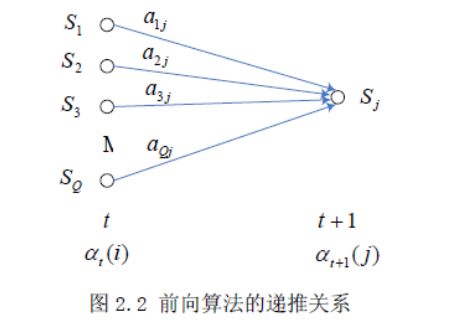与前向算法相似，后向算法是从后向前递推计算输出概率的方法。定义后向概率变量$$\beta_t(i) : \beta_t(i) = P(o_{t+1},o_t,\cdots,o_T | q_t = S_i,\lambda)$$ 则$\beta_t(i)$可由下面递推公式计算，后向算法的递推关系图如下所示: 初始化 :$\beta_T(i) = 1, 1 \leq i \leq Q$递推公式:$$\beta_t(i) = \sum_{j=1}^Q a_{ij} b_j(o_{t+1}) \beta_{t+1}(j), 1 \leq i \leq Q,t = T-1,\cdots,1$$ 结束 :$P(O | \lambda) = \sum_{i=1}^Q \beta_1(i) \pi_i = \beta_0(1)$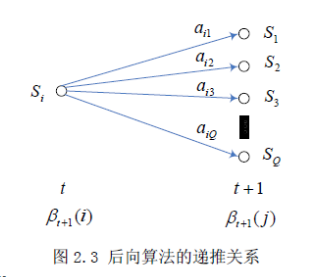根据前向变量和后向变量的定义，显然下式成立 ： $$P(O | \lambda) = \sum_{i=1}^Q \sum_{j=1}^Q \alpha_t(i) a_{ij} b_j(o_{t+1}) \beta_{t+1}(j),1 \leq t \leq T-1$$ 如何来理解该式子？$\alpha_t(i) a_{ij} b_j(o_{t+1}) \beta_{t+1}(j)$表示$t$时刻为状态$q_i$的前向概率（根据前向概率的定义，已经计算了$t$时刻状态为$q_i$时观测到$o_t$的概率），$t$时刻状态$q_i$转移到$t+1$时刻的状态$q_j$，在$t+1$时刻状态$q_j$下观测到$o_{t+1}$的概率，最后乘以$t+1$时刻状态为$q_j$的后向概率（因为按后向概率的定义不包含当前状态的观察概率$b_j(o_{t+1})$的计算）；分别求所有可能的状态$q_i,q_j$的组合，就是$P(O|\lambda)$. 利用该算法减少计算量的原因在于每一次计算直接引用前一个时刻的计算结果，避免重复计算.这样，利用前向概率计算$P(O|\lambda)$的计算量是$O(N^2T)$阶的，而不是直接计算的$O(TN^T)$阶. ### 监督学习方法 对于$\text{HMM}$参数估计问题，可按训练数据的特点来分是有监督学习还是无监督学习，如果是有监督学习的话，可直接通过对训练样本做一些统计即可得到$\text{HMM}$的参数估计；在中文自然语言处理中，许多标注问题就是这么做的. 假设已给训练数据包含$K$个长度相同的观察序列和对应的状态序列${(O_1,Q_1),(O_2,Q_2),\cdots,(O_K,Q_K)}$，那么我们可以直接利用极大似然估计法来估计隐马尔科夫模型的参数，具体如下: 转移概率$a_{ij}$的估计设样本中时刻$t$处于状态$q_i$，时刻$t+1$时刻由状态$q_i$转移到了$q_j$，此种情况出现的频数为$A_{ij}$，那么转移概率$a_{ij}$的估计值为 $$\hat{a}_{ij} = \frac{ A_{ij} }{\sum_{j=1}^Q A_{ij} },i = \{S_1,S_2,\cdots,S_Q\};j = \{S_1,S_2,\cdots,S_Q\}$$ 即统计由状态$q_i$转移到状态$q_j$占状态$q_i$转移出去的比例. 观测概率$b_{q_j}(v)$的估计设样本中状态为$q_j$并观测为$o_v$的频数是$B_{jv}$，那么状态为$q_j$观测为$o_v$的概率$b_{q_j}(v)$的估计是: $$\hat{b}_{q_j}(v) = \frac{B_{jv} }{\sum_{v=1}^M B_{jv} }$$ 其中，$j = 1,2,\cdots,T;\quad v = 1,2,\cdots,M$. ### Baum-Welch 算法 对于无监督学习的 HMM 参数估计问题，可以使用 Baum-Welch算法. 首先定义两个变量$r_t(i)$和$\xi_t(i,j)$，给定观察序列$O(e_1,e_2,\cdots ,e_T)$和模型参数$\lambda=\{A,B,\pi \}$; •$r_t(i)$为系统在时刻$t$系统处在状态$S_i$的概率，即$$r_t(i) = P(q_t = S_i | O,\lambda)$$ •$\xi_t(i,j)$为$t$时刻状态为$S_j$，到$t+1$时刻系统状态转为$S_j$的概率，即$$\xi_t(i,j) = P(q_t = S_i,q_{t+1} = S_j | O,\lambda)$$ 根据前向-后向算法中$\alpha_t(i)$和$\beta_t(i)$定义有: $$r_t(i) = \frac{\alpha_t(i) \beta_t(i)}{\sum_{j=1}^Q \alpha_t(j) \beta_t(j)},1 \leq i \leq Q \\ \xi_t(i,j) = \frac{\alpha_t(i) a_{ij} b_j(e_{t+1}) \beta_{t+1}(j)}{P(O|\lambda)} \\ \qquad =\frac{\alpha_t(i) a_{ij} b_j(e_{t+1}) \beta_{t+1}(j)}{\sum_{m=1}^Q \sum_{n=1}^Q \alpha_t(m) a_{mn} b_n(e_{t+1}) \beta_{t+1}(n)} ,1 \leq i,j \leq Q$$ 则对于观察序列$O(e_1,e_2,\cdots ,e_T)$而言，系统所处于状态$S_j$的总次数（期望值）为$\sum_{i=1}^Q r_t(i)$；同样，系统从状态$S_i$转移到$S_j$的总次数为$\sum_{i=1}^Q \xi_t(i,j)$.假设给定训练数据只包含$K$个长度为$T$的观测序列$\{O_1,O_2,\cdots,O_K \}$而没有对应的状态序列，目标是学习隐马尔科夫模型$\lambda=\{A,B,\pi \}$的参数.我们将观测序列数据看作观测数据$O$，状态序列数据看作不可观测的隐数据$I$，那么隐马尔科夫模型事实上是一个含有隐变量的概率模型 $$P(O|\lambda) = \sum_{I} P(O|I,\lambda) P(I|\lambda)$$ 它的参数学习可以用EM算法来实现. 1. 确定完全数据的对数似然函数所有观测数据写成$O = (o_1,o_2,\cdots,o_T)$，所有隐数据写成$I = (i_1,i_2,\cdots,i_T)$，完全数据是$(O,I) = (o_1,o_2,\cdots,o_T,i_1,i_2,\cdots,i_T)$，完全数据的对数似然函数是$\log(P(O,I|\lambda))$2. EM 算法的 E 步: 求$Q$函数$Q(\lambda,\overline{\lambda})$$Q(\lambda,\overline{\lambda}) = \sum_{I} \log \left( P(O,I|\lambda) P(O,I|\overline{\lambda}) \right)$$其中，$\overline{\lambda}$ 是隐马尔科夫模型参数的当前估计值，$\lambda$ 是要极大化的隐马尔科夫模型参数.$$P(O,I|\lambda) = \pi_{i_1} b_{i_1}(o_1) a_{i_1 i_2} b_{i_2}(o_2) \cdots a_{i_{T-1} i_T} b_{i_T}(o_T)$$于是函数 $Q(\lambda,\overline{\lambda})$ 可以写成:$$Q(\lambda,\overline{\lambda}) = \sum_{I} ( \log \pi_{i_1} ) P(O,I|\overline{\lambda}) \\ \sum_{I} ( \sum_{t=1}^{T-1} \log a_{i_t i_{t+1}} ) P(O,I|\overline{\lambda}) + \sum_{I} ( \sum_{t=1}^T \log b_{i_t}(o_t) ) P(O,I|\overline{\lambda})$$式中求和都是对所有训练数据的序列总长度 $T$ 进行的.

1. EM 算法的 M 步: 极大化 $Q$ 函数 $Q(\lambda,\overline{\lambda})$ 求模型参数 $A,B,\pi$由于要极大化的参数在 $Q(\lambda,\overline{\lambda})$ 中单独地出现在 3 个项中，所以只需对各项分别极大化.

(1). 第一项可以写成:$$\sum_{I} ( \log \pi_{i_1} ) P(O,I|\overline{\lambda}) = \sum_{i=1}^N (\log \pi_i) P(O,i_1 = i | \overline{\lambda})$$注意到 $\pi_i$ 满足约束条件$\sum_{}^N \pi_i = 1$ ，利用拉格朗日乘子法，写出拉格朗日函数:$$L(\pi_i,\gamma) = \sum_{i=1}^N (\log \pi_i) P(O,i_1 = i | \overline{\lambda}) + \gamma ( \sum_{i=1}^N \pi_i - 1 )$$对其求偏导数并令结果为零:$$\frac{\partial L}{\partial \pi_i} = \frac{1}{\pi_i} P(O,i_1 = i | \overline{\lambda}) + \gamma = 0 \\ P(O,i_1 = i | \overline{\lambda}) + \gamma \pi_i = 0 \\ \gamma = -P(O|\overline{\lambda}) \\ \pi_i = \frac{P(O,i_1 = i | \overline{\lambda})}{P(O|\overline{\lambda})}$$

(2). 第 2 项可以写成:$$\sum_{I} ( \sum_{t=1}^{T-1} \log a_{i_t i_{t+1}} ) P(O,I|\overline{\lambda}) \\ = \sum_{i=1}^N \sum_{j=1}^N \sum_{t=1}^{T-1} (\log a_{ij}) P(O,i_t = i,i_{t+1} = j|\overline{\lambda})$$

(3). 第 3 项与第 2 项的计算类似:$$\sum_{I} \left( \sum_{t=1}^T \log b_{i_t}(o_t) \right) P(O,I|\overline{\lambda}) = \sum_{j=1}^N \sum_{t=1}^T \log b_j(o_t) P(O,i_t = j | \overline{\lambda})$$同样用拉格朗日乘子法，约束条件是 $\sum_{k=1}^M b_j(k) = 1$ .注意，只有在 $o_t=v_k$ 时 $b_j(o_t)$ 对 $b_j(k)$ 的偏导数才不为 $0$，以 $I(o_t=v_k)$ 表示，求得$$b_j(k) = \frac{\sum_{t=1}^T P(O,i_t = j | \overline{\lambda}) I(o_t = v_k)}{\sum_{t=1}^T P(O,i_t = j | \overline{\lambda})}$$

#### Note

• 初始化对 $n=0$，选取 $a_{ij}^{(0)},b_j(k)^{(0)},\pi_i^{(0)}$，得到模型 $\lambda^{(0)} = (A^{(0)},B^{(0)},\pi^{(0)})$
• 递推，对 $n = 1,2,\cdots$$a_{ij}^{(n+1)} = \frac{\sum_{t=1}^{T-1} \xi_t(i,j)}{\sum_{t=1}^{T-1} \gamma_t(i)} \\ b_j(k)^{(n+1)} = \frac{\sum_{t=1,o_t = v_k}^T \gamma_t(j)}{\sum_{t=1}^T \gamma_t(j)} \\ \pi_i^{(n+1)} = \gamma_1(i)$$右端各值按观测$O = (o_1,o_2,\cdots,o_T)$和模型$\lambda^{(n)} = (A^{(n)},B^{(n)},\pi^{(n)})$计算. • 终止，得到模型参数$\lambda^{(n+1)} = (A^{(n+1)},B^{(n+1)},\pi^{(n+1)})$## R中的HMM ]]> 隐马尔可夫模型（Hidden Markov Model，$\text{HMM}$）是统计模型，它用来描述一个含有隐含未知参数的马尔可夫过程。其难点是从可观察的参数中确定该过程的隐含参数。然后利用这些参数来作进一步的分析，例如模式识别。 概率论（离散空间）杂谈 http://ulsonhu.cn/概率论杂谈篇一.html 2018-03-19T16:00:00.000Z 2018-03-28T11:22:20.487Z 关于概率论与统计学的不同，相信学过统计学的同学，看过上图后一定能秒懂。 统计学：根据手中信息，猜猜桶里有啥？ 概率论：根据桶中信息，猜猜手里有啥？ ## 概率空间 所有基本事件组成的集合，其中的每一个基本事件又称做样本点，样本空间用$\boldsymbol {\Omega }$表示，样本点用$\omega$表示,$\Omega=\{\omega \}$1.$\mathcal{\Omega}$－Sample space 样本空间，试验中所有可能结果的集合。（注：每个结果需要互斥，所有可能结果必须被穷举） 2.$\mathcal{F}$－Set of events 事件集合，是$\Omega$的一些子集构成的集合，并且它需要满足以下三点特性（也就是必须是$\sigma$－field）： •$\mathcal{\Phi \in F}$（也就是必须包含不可能事件） • 如果$E\in F,E^C \in \mathcal{F}$。 • 如果$E_1,E_2,\cdots ,E_i \in \mathcal{F}$,那么$U_{i=1}^{\infty}E_i \in \mathcal{F}$1.$\mathcal{P}$－Probability measure 概率测度（或概率），描述一次随机试验中被包含在$\mathcal{F}$中的所有事件的可能性。并且它「碰巧」也需要满足三点特性： •$0\leq P(E_i)\leq 1$（实际限制了总测度为1） •$P(\Omega)=1$（包含样本空间并且概率为1） • 如果$E_1,E_2,\cdots ,E_i$是互斥事件,那么$P\{U_{i=1}^{\infty} E_i \}=\sum_{i=1}^{\infty} E_i$## 谈斯特林公式 $$n! \sim \sqrt{2\pi }n^{n+\frac{1}{2} }e^{-n}$$ 证明见附录，这是概率论中非常重要的理论，基于此可以得到一些精确的数值估计 ### Stirling与概率问题 什么是一个事件（event）的概率？这是概率论最基本也是争议最多的问题【例】：抛一枚硬币，出现正面的概率？ 这是什么意思，通常认为，将这枚硬币独立的抛「很多次」，那么正面出现的次数「大约」占一半。这是在随机事件中很确定的事。所谓的「平均律」(The law of average)或「大数定律」(The law of large numbers)隐约也是在解释这个说法。现在我们从常识出发，来考虑数学表达。 【问题1】抛$2n$次硬币，正面恰好出现$n$次的概率又多大？根据组合学，抛$2n$次硬币，共有$2^{2n}$种可能，假设每一种发生的结果机会均等，那么$2n$次中有$n$次正面的结果共有$C_{n}^{2n}$种，故得概率为$p_{2n} = \frac{C_n^{2n}}{2^{2n}} = \frac{(2n)!}{2^{2n}\cdot n!\cdot n!} \qquad (1)$更有趣的是，当$n$趋向$\infty$时，$p_{2n}$会趋近多少？上述常识性的解释似乎是说，$\lim_{n \to \infty } p_{2n} = 1$，这成立吗？这需要对$(1)$式作精确的估计，于是引出下面的问题 【问题2】当$n$很大时，如何估算？明确地说：当$n$趋向$\infty$时，$n!$的渐进相等式（Asymptotically equal formula）是什么？ 即要找一个${a_n}$使得 $$\lim_{n \to \infty} \frac{n!}{a_n} = 1,\quad n! \sim a_n$$ 我们希望找到这样的${a_n}$，然后带入$(1)$式中计算极限值$\lim_{n \to \infty} p_{2n}$，就可以检验上述常识性的说法是否正确。$n!$的渐进相等式（Asymptotically equal formula）存在吗？如何找？这时候就需要Stirling公式出场了。 ### 初步否定常识性的概率 现在我们要利用Stirling 公式来探讨概率之谜(the enigma of probability)。 首先观察到一个显然的 【补题】设$\{a_n\},\{b_n\},\{c_n\}$及$\{d_n\}$皆为正项数列且$\lim_{n \to \infty} \frac{a_n}{b_n} = l$。若$a_n \sim c_n$且$b_n \sim d_n$，则$\lim_{n \to \infty} \frac{c_n}{d_n} = l$。接着计算「丢$2n$次硬币，恰好出现$n$次正面的机率$p_{2 n}$在$n \to \infty$的极限」 $$\lim_{n \to \infty}p_{2n} = \lim_{n \to \infty} \frac{(2n)!}{2^{2n}n!n!} \\=\lim_{n \to \infty} \frac{\sqrt{2\pi 2n}(2n)^{2n}e^{-2n}}{2^{2n}\cdot 2\pi \cdot n\cdot n^{2n}\cdot e^{-2n}} \\=\lim_{n \to \infty}\frac{1}{\sqrt{\pi n}}=0$$ 【定理3】$ \lim_{n \rightarrow \infty} p_{2n} = 0$。因此，当$n \to \infty$时，$p_{2n}$不但不如原先预计的趋近于1（即必然发生），反而是趋近于0（即不可能发生）。这警告我们，概率的解释与经验有很大出入。 【常识性的说法】「丢很多次硬币正面大约占一半。」如果将「大约占一半」， 解释为「恰好是一半」的说法，点估计显然是不对的。如何修正呢？自然想到的是改用区间估计的说法。 为了叙述方便起见，我们引入随机变量(random variable)的概念。对于$k =1, 2, 3, \cdots $，令随机变量 $$f(n) =\begin{cases}\epsilon_k = 1, & \text{第K次硬币得到正面 } \\\epsilon_k = 0, & \text{第K次硬币得到反面}\end{cases}$$ 再令$$S_n = \epsilon_1 + \epsilon_2 + \cdots + \epsilon_n$$ 这也是一个随机变数，定义在某个机率空间$\mathcal{(\Omega, F , P )}$上，代表丢$n$次硬币中，正面出现次数之随机变数，它具有二项分布。于是「$S_{2 n} = n$」就表示丢$2n$次正面恰好出现$n$次的事件，其概率记为$P ( S_{2 n} = n )$。 因此【定理3】是说。$\lim_{n \rightarrow \infty} P(S_{2n}=n)=0$进一步我们猜想：$S_{2n}$落在$n$的附近之概率应该会大起来吧？ 也许这是「正面大约占一半」更贴切的解释。精确的计算是探求隐晦奥秘的不二法门，让我们就来算算看。 令$a > 0​$唯一个固定数，那么 $$P(n-a \leq S_{2n} \leq n+a) = \sum_{k=n-a}^{n+a} C_{k}^{2n}\cdot \frac{1}{2^{2n} } \\ \sim \frac{2a}{ \sqrt{\pi n}} \rightarrow 0 ,when \quad n \rightarrow \infty$$ 因此我们又得到一个不出所料的结果： 【定理4】：对任意固定数$a > 0$，$$\lim_{b \to \infty} P( \mid S_{2n} - n \mid \leq a ) = 0$$ 换言之，以$n$为中心，左右之长皆为$a$之区间，概率依然为零！另外，将偶数$2n$改成奇数$2 n +1$，【定理4】仍然成立。因为当$n \to \infty$时，相应项的比值为 $$\frac{ C_{k}^{2n+1}\cdot \frac{1}{ 2^{2n+1} } }{C_{k}^{2n} \frac{1}{2^{2n}}} = \frac {2n+1}{2n+1-k} \cdot \frac{1}{2} \to \frac{1}{2}$$所以$$P( -a \leq S_{2n+1} - \frac{2n+1}{2} \leq a ) \\ = \sum_{k=-a+ \frac {2n+1}{2}}^{a+ \frac {2n+1}{2}} C_k^{2n} \frac{1}{2^{2n+1}}\\\sim \frac{1}{2}\sum_{k=-a+ \frac {2n+1}{2}}^{a+ \frac {2n+1}{2}} C_k^{2n} \frac{1}{2^{2n}} \to 0$$ 所以得到【定理5】：对任意有限正数$a$，恒有$$\lim_{n \to \infty} P(-a \leq S_{2n+1} - \frac{2n+1}{2} \leq a) = 0$$ 将上述【定理4】与【定理5】归结起来就得到： 【定理6】：对任意有限正数$a$，恒有 $$\lim_{n \to \infty} P(-a \leq S_n - \frac{n}{2} \leq a) = 0$$ 这是一个令人惊异的结果，但也令人失望！逻辑的闷棍把常识的观点打得眼冒金星。用任何有限区间$[- a , a\ ]$来网罗住$S_n - \frac{n}{2}$所散布的概率，当$n \to \infty$时，根本没有往到任何概率，概率全部流失掉！ 换言之，丢$n$次，出现正面的次数，落在包含$\frac{n}{2}$的任何有限区间的机会，当$n$很大时，微乎其微。 什么是概率？它仍然是很神秘！ 1. 表面来看，Stirling 公式并不漂亮，但却很多用途，它是揭开许多深刻奥秘的钥匙。在研究二项分布的性质时，De Moivre 最先得到这个公式（1718年）；后来James Stirling 在1730年又重新得到它。 1.$n!$的连续变化就是Gamma函数，内容精彩丰富，这是Euler的贡献。 ## 附录 斯特林公式证明，相当于一道考研题目 $$log{n!}=log{1}+log{2}+\cdots+log{n}$$ 因为$\log x$为单调函数，所以 $$\int_{k-1}^{k} \log{x} dx < \log{n!} < \int_{k}^{k+1}\log{x}dx$$把上式对$k$从$1$到$n$相加得到 $$\int_{0}^{n}\log{x}dx < \log{n!} < \int_{1}^{n+1}\log{x}dx \qquad(1)$$$$\Leftrightarrow n\log{n}-n < \log{n!} < (n+1)\log{(n+1)}-n \qquad(2)$$这是一个双重不等式，提示我们把$\log {n!}$与两端数的算数平均做比较，即想到$(n+\frac{1}{2})\log{n}-n$$d_n=\log{n!}-(n+\frac{1}{2})\log{n}+n \qquad (3)$$$$d_n - d_{n+1}=\log{n!}-(n+\frac{1}{2})\log\frac{n+1}{n}-1$$$$\frac{n+1}{n}=\frac{1+\frac{1}{2n+1}}{1-\frac{1}{2n+1}}$$$\boldsymbol{Taylor}$展开得$$d_n - d_{n+1}=\frac{1}{3(2n+1)^2}+\frac{1}{5(2n+1)^4}+\cdots$$把上式与一个公比的几何级数进行比较得$$0 < d_n-d_{n+1} < \frac{1}{3\left [ (2n+1)^2-1 \right ]}=\frac{1}{12n}-\frac{1}{12(n+1)}$$知是${d_n}$下降序列，说明序列${d_n-(12n)^{-1} }$是上升的，因此，极限$\lim d_n = C$存在且有限，但是，由$(3)$知：$d_n \rightarrow C$等价于$$n! \sim e^C \cdot n^{n+\frac{1}{2} } \cdot e^{-n}$$

]]>

通常做数据分析&挖掘的workflow：拿到数据之后先要做一个数据的预处理(pandas+numpy+scipy)，接着会要对数据包含的特征做一些可视化输出(matplotlib)，之后需要提取特征&建模调参(numpy+scikit-learn)，有了模型与结果，最后归纳整理做presentation & report.

## Numpy

FunctionUseage
array.shape查看或改变数组的大小
array.reshape修改数组的尺寸，原数组不变，两个数组共享内存，如果修改值的话这两个数组都会变
array.size数组元素的总个数，等于shape属性中元组元素的乘积
array.ndim数组的维数
array.dtypearray的数据规格
numpy.zeros(dim1,dim2)创建dim1*dim2的零矩阵
numpy.eye(n)创建$n*n$单位矩阵
numpy.identity(n)创建$n*n$单位矩阵
numpy.arange类似于list的range函数，通过指定初始值，终值，和步长来生成一维数组
array.astype(numpy.float64)更换矩阵的数据形式
array * array矩阵点乘
array[a:b]切片
array.copy()得到ndarray的副本，而不是视图
array [a] [b]=array [ a, b ]两者等价
data[[4,3,0,6]]索引，将第4,3,0,6行摘取出来，组成新数组
numpy.reshape(a,b)将ab的一维数组排列为ab的形式
array([a,b,c,d],[d,e,f,g])返回一维数组，分别为[a,d],[b,e],[c,f],[d,g]
array.Tarray的转置
numpy.random.randn(a,b)生成$a*b$的随机数组
numpy.dot(matrix_1,matrix_2)矩阵乘法
array.transpose( (1,0,2,etc.) )对于高维数组，转置需要一个由轴编号组成的元组

numpy库提供了matrix类，使用matrix类创建的是矩阵对象，它们的加减乘除运算缺省采用矩阵方式计算。但是由于NumPy中同时存在ndarray和matrix对象，因此很容易将两者弄混。

## Pandas

1. 汇总和计算描述统计，处理缺失数据 ，层次化索引
2. 清理、转换、合并、重塑、GroupBy技术
3. 日期和时间数据类型及工具

## Matplotlib

Python中最著名的绘图系统，很多其他的绘图例如Seaborn（针对pandas绘图而来）也是由其封装而成。其中针对不是很复杂的数据，推荐使用Seaborn，容易上手，图表美观。Seaborn参考

1. 散点图，折线图，条形图，直方图，饼状图，箱形图的绘制。
2. 绘图的三大系统：pyplot，pylab(不推荐)，面向对象
3. 坐标轴的调整，添加文字注释，区域填充，及特殊图形patches的使用
4. 金融的同学要注意的是：可以直接调用Yahoo财经数据绘图（很好用）

### 简单的散点图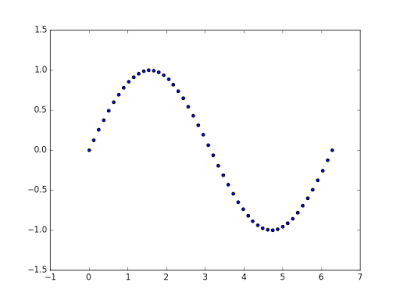### 使用子图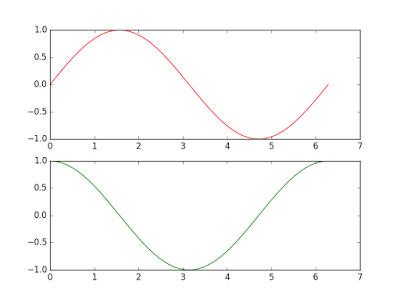### 添加标题，坐标轴标记和图例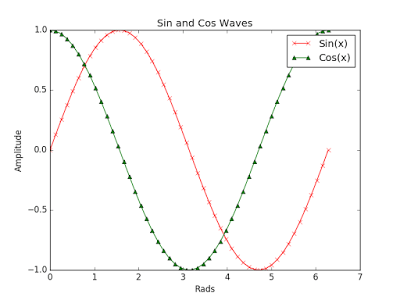]]>
<p>用python做数据分析与科学计算主要涉及Numpy, Pandas, Scipy, Scikit-learn, Scipy等库，工作环境为Anaconda，这个IDE集成了大部分常用的包。同时，可以实现本地环境下Python的多版本切换，可参考之前文章,<a href="https://ulsonhu.github.io/MacOS%E4%B8%8B%E4%BD%BF%E7%94%A8python%E7%9A%84%E5%A4%9A%E7%89%88%E6%9C%AC%E6%96%B9%E6%A1%88.html" target="_blank" rel="noopener">MacOS下使用python的多版本方案</a></p>
SAS基础学习篇(一) http://ulsonhu.cn/SAS基础学习篇(一).html 2018-03-13T16:00:00.000Z 2018-03-21T02:59:56.496Z 参考书籍

1. 《SAS编程与数据挖掘商业案例》（预热）
2. 「the Little SAS Book」(推荐)
3. 「Applied Econometrics Using The SAS System」

SAS编程主要包括DATAPROC两部分

## 基本函数

SAS文本类函数
ANYALNUM(arg,start)返回第一次出现任意数字或字母的位置，可选开始位置start
ANYALPHA(arg,start)返回第一次出现任意字母的位置，可选开始位置start
ANYDIGIT(arg,start)返回第一次出现任意数字的位置，可选开始位置start
ANYSPACE(arg,start)返回第一次出现任意空白的位置，可选开始位置start
CAT(arg1,arg2,…argn)连接字符串，留下头尾空白
CATS(arg1,arg2,…argn)连接字符串，删除头尾空白
CATX(‘separator-string’, arg-1,…,arg-n)连接字符串，删除头尾空白并用指定标点连接
COMPRESS(arg, ‘char’)移除字符串中的空格和可选字符
INDEX(arg, ‘string’)返回指定字符在变量中的位置
LEFT(arg)字符串左对齐
LENGTH(arg)返回字符串长度，不考虑尾部空格
PROPCASE(arg)首字母大写
SUBSTR(arg,position,n)从字符串中提取指定开始位置指定长度字符
TRANSLATE(source,to1,from1,…ton,fromn)替换字符
TRANWRD(source,from,to)替换字符串
TRIM(arg)删除尾部空白
UPCASE(arg)替换成大写
SAS数值函数
INT(arg)返回整数
LOG(arg)自然对数
LOG10(arg)$10$为底对数
MAX(arg1,arg2,…argn)最大值
MEAN(arg1,arg2,…argn)均值
MIN(arg1,arg2,…argn)最小值
N(arg1,arg2,…argn)非缺失值个数
NMISS(arg1,arg2,…argn)缺失值个数
ROUND(arg, roundoffunit)保留几位小数
SUM(arg1,arg2,…argn)求和
SAS日期函数
DATEJUL(julian-date)标准julian日期到SAS日期
DAY(date)返回「日」
MDY(month,day,year)年月日到SAS日期
MONTH(date)返回「月」
QTR(date)返回季度
TODAY()今日
WEEKDAY(date)返回周几（周日为1）
YEAR(date)返回「年」
YRDIF(start-date,end- date,’ACTUAL’)返回相差年份

## 基本模块调用

### PROC的基本内容：CONTENT

PROC:content，可以显示数据集的主要特性。比如，

### SAS PROC里面改变输出格式：FORMAT

• 文本型：$HEXw.$w.
• 日期型：DATEw.（输出为ddmmyy或者ddmmyyyy）、DATETIMEw.d（输出为ddmmyy:hh:mm:ss）、DAYw.（输出为dd）、EURDFDDw.JULIANw.MMDDYYw.（输出为mmddyy或mmddyyyy）、TIMEw.d（输出为hh:mm:ss）、WEEKDATEw.（输出为工作日）、WORDDATEw.(输出为单词)。
• 数字型：BESTw.（自动选择）、COMMAw.d（逗号分隔）、DOLLARw.d（货币）、Ew.（科学计数法）、PDw.dw.d（标准小数）

### SAS总结数据:MEANS

$SAS$当然还有类似于$Excel$的数据透视表和$R$的data.table的模块，就是MEANS。可以输出的描述性统计值，包括最大值、最小值、平均值、中位数、余非缺失值个数、缺失值个数、范围、标准差、和等等。此外，还可以使用BY或者CLASS进行分组统计，VAR选择变量等。

SAS代码：

### SAS PROC汇报表格：TABULATE

SAS代码：

BOSS级汇报表格呈现了…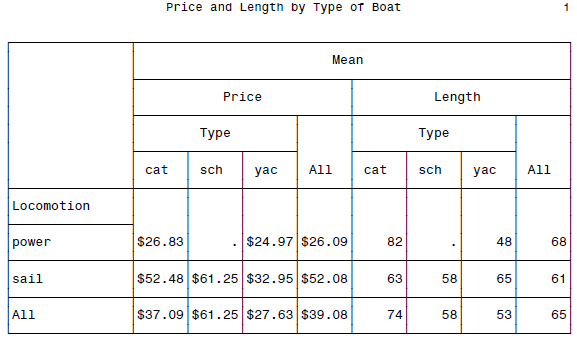SAS里面的报告：REPORT还有一个REPORT，看到有TABULATE的时候我已经不奇怪并略略的有些期待一个做报告的模块出现了。这东西基本就是前面几个的超级混合体，反正你想搞到的汇报模式总是能够搞出来的。

### SAS数据总结综述

]]>

Excel知识点备忘 http://ulsonhu.cn/Excel知识点备忘.html 2018-03-11T16:00:00.000Z 2018-03-23T15:52:50.368Z Excel系统学习：视频教程

## 相对引用与绝对引用

• $A1列绝对引用 • A$1行绝对引用
• $A$1行列都为绝对引用

• 一般排序
• 多条件排序
• 按照特定字段排序
• 排序的延伸

## 一、时间与日期函数

1. today()返回日期格式的当前日期,输入日期快捷键Ctrl+;
2. now(),输入日期时间快捷键Ctrl+Shift+;
3. weekday(serial_number,return_type)
4. workday()
5. dateif(start_date,end_date,unit[Y|M|D])返回两个日期之间年/月/日的间隔数

## 二、统计函数

1. count
2. countif(range,criteria)计算区域中满足指定条件的单元格个数
3. countifs(range1,criteria1,range2,criteria2,...)
4. sumif(range,criteria,sum_range)
5. sumifs(sum_range,criteria_range1,criteria1,criteria_range2,criteria2,...)

## 三、查找和引用函数

1. vlookup(lookup_value,table_array,col_index_num,range_lookup)
• lookup_value需要在数据表第一列查找的数值
• table_array需要查找的数据区域
• col_index_num为待返回的匹配值的列序号
• range_lookup精确匹配与模糊匹配

### 关于跨表引用

AP11001老婆饼老婆饼（花生）6.5
AP11002老婆饼老婆饼（桂花）6.5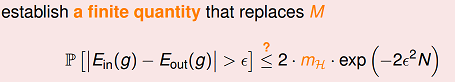## Effective Number of Hypotheses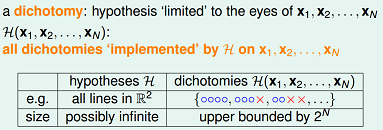注意到，如果对平面上的4个点来分类，根据前面分析，输出的结果向量只有14种可能，即有14个dichotomies。如果有$N$个样本数据，那么有效的假设个数定义为： $effective(N) = H$作用于样本集$D$“最多”能产生多少不同的dichotomy。所以有一个直观思路，能否用$effective(N)$来替换Hoeffding不等式中的M。接下来我们来分析下$effective(N)$。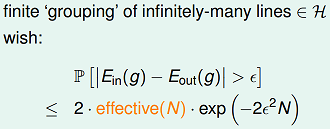## Growth Function

$H$作用于$D$“最多”能产生多少种不同的dichotomies？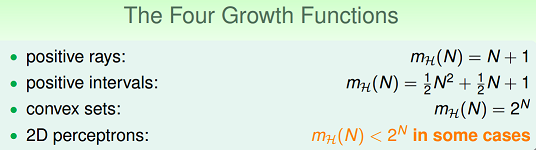对于第一个例子，positive ray，相当于是正向的射线。该假设空间，作用于1个样本点，可以产生2种dichotomies：(–1)，(+1)。作用于2个样本点，可以产生3种dichotomies：(–1,+1)，(–1,–1)，(+1,+1)。作用于3个样本点，可以产生4种dichotomies。依次类推，可以推导出其成长函数 $m_H(N)=N+1$；求解出$m_H(N)$后，那是不是可以考虑用$m_H(N)$替换$M$? 如下所示：$$P[|E_{in}(g)-E_{out}(g)|>\epsilon]\leq 2\cdot m_H(N)\cdot N$$

## Break Point与Shatter

Shatter的概念：当假设空间H作用于$N$个input的样本集时，产生的dichotomies数量等于这$N$个点总的组合数$2N$是，就称：这$N$个inputs被$H$给shatter掉了。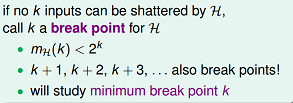## VC Bound

• $B(2,2)=3$。因为break point=2，任意两个点都不能被shatter，$m_H(2)$肯定小于$2^2$，所以$B(2,2)=3$。
• $B(3,2)=4$。因为任意两个点都不能被shatter，那么3个点产生的dichotomies不能超过4，所以$B(3,2)=4$。
• $B(N,1)=1$。
• $B(N,k)=2^N \quad for \quad N < k$;$\qquad B(N,k)=2^{N–1} \quad for \quad N=k$；
• $B(4,3)=？$去掉其中的一个数据点$x_4$后，考虑到break point=3，余下数据$(x1,x2,x3)$的dichotomies数目不能超过$B(3,3)$。当扩展为$(x1,x2,x3,x4)$时，$(x1,x2,x3)$上的dichotomies只有部分被重复复制了，设被复制的dichotomies数量为$a$，未被复制的数量为$b$。于是有$B(3,3) = a+b; B(4,3) = 2a + b$。因为$a$被复制了，表示$x_4$有两个取值，那么$(x1,x2,x3)$上的$a$应该小于等于$B(3,2)$。所以推导出$B(4,3) = 2a + b \leq B(3,3) + B(3,2)$。对于任意$N>k$，类推可以得到，$B(N,k) \leq B(N−1,k)+B(N−1,k−1)$

OK，到目前为止，关于$m_H(N)$的推导结束。回到growth function小节提出的问题，能否用$m_H(N)$直接替换M?

## VC dimension

• $H$的$d_{vc}$是有限的，这样VC bound才存在。(good H)；$N$足够大(对于特定的$d_{vc}$而言)，这样才能保证VC bound不等式的bound不会太大。(good D)

]]>